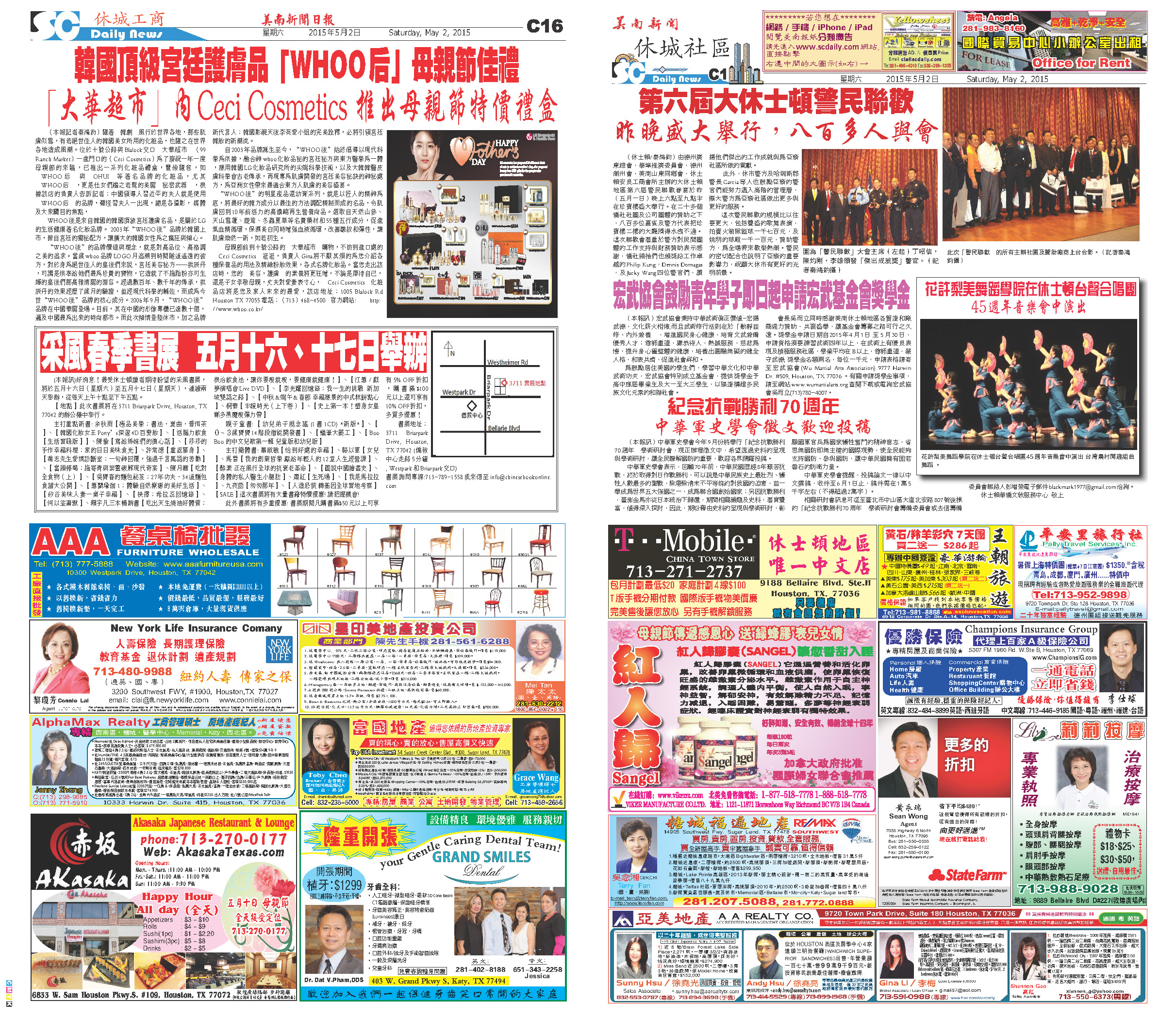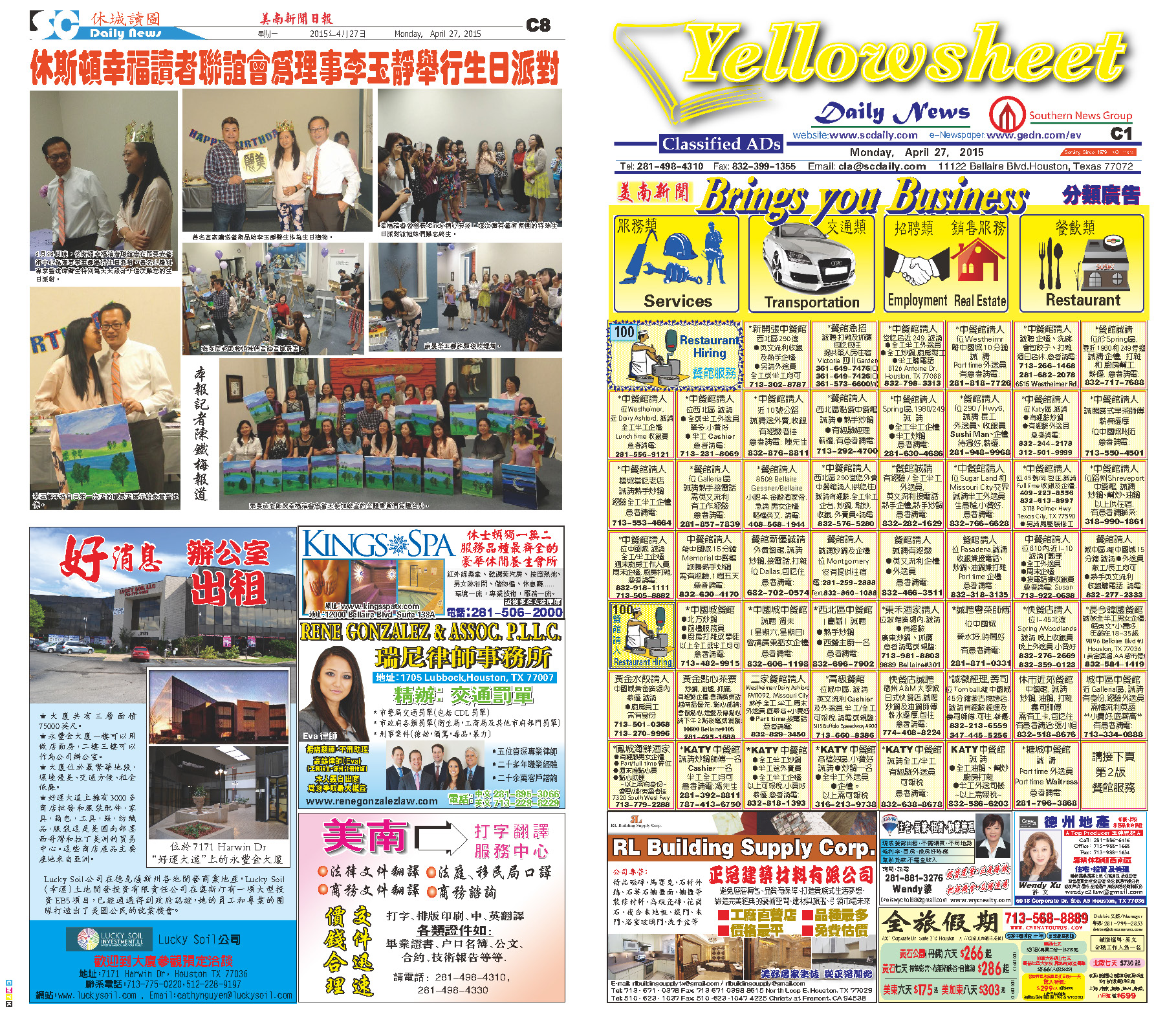150503 Epaper

 A Section B SectionC Section150502 Epaper

 A Section B SectionC Section D Section150501 Epaper

 A Section B SectionC Section D Section150430 Epaper

 A Section B SectionC Section D Section150429 Epaper

 A Section B SectionC Section D Section150428 Epaper

 A Section B SectionC Section D Section150427 Epaper

 A Section B SectionC Section D Section150426 Epaper

 A Section B SectionC Section D Section150424 Epaper

 A Section B SectionC Section D Section150425 Epaper

 A Section B SectionC Section D Section150423 Epaper

 A Section B SectionC Section D Section